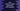# Java ArrayList addAll method explanation with examples## Java ArrayList addAll method explanation with examples:

addAll method of Java ArrayList can be used to add multiple elements to an ArrayList. It has two variants. We can add all items of a collection to the end of an ArrayList or we can add the items from a specific index.

In this post, we will learn how to use this method with examples.

This method is defined as like below:

``public boolean addAll(Collection<? extends E> c)``
• It appends all elements of the collection c to the end of this ArrayList.
• It maintains the same order as specified by the iterator of the collection.
• The behavior of the operation is undefined if the collection is modified when the process is in process.

• It returns a boolean value. It returns true if the list is changed for this call. Else, it returns false.

#### Exception:

It can throw NullPointerException if the collection we are passing is null.

### Example program:

Let’s take a look at the below program:

``````import java.util.ArrayList;
import java.util.Arrays;

class Main {
public static void main(String[] args) {
ArrayList<Integer> firstArrayList = new ArrayList<>(Arrays.asList(1, 2, 3, 4, 5));
ArrayList<Integer> secondArrayList = new ArrayList<>(Arrays.asList(6, 7, 8, 9, 10));

System.out.println("firstArrayList after the change: " + firstArrayList);
}
}``````

Here,

• firstArrayList and secondArrayList are two arraylists of integers.
• We are using addAll to add the contents of secondArrayList to the end of firstArrayList.
• The last line is printing the contents of firstArrayList.

If you run this program, it will print the below output:

``firstArrayList after the change: [1, 2, 3, 4, 5, 6, 7, 8, 9, 10]``

As you can see here, it appended the content of secondArrayList to the end of the firstArrayList.

This method is similar to the above one. The only difference is that we can also pass the index as the first parameter. The insertion will start from this index. The other elements will be shifted to the right. The order of the ArrayList will be same as it is returned by their iterator.

Below is the definition of this method:

``public boolean addAll(int index, Collection<? extends E> c)``
• index is the index to start the insertion.
• c is the collection to add.

#### Return value:

It returns true if the list is changed by this method call.

#### Exceptions:

It can throw IndexOutOfBoundsException or NullPointerException.

• It will throw IndexOutOfBoundsException if the provided index is invalid, i.e. if it is less than 0 or if it is greater than the length of the ArrayList where we are appending the new collection.
• It will throw NullPointerException if the collection we are passing is null.

### Example program:

Let’s take a look at the below program:

``````import java.util.ArrayList;
import java.util.Arrays;

class Main {
public static void main(String[] args) {
ArrayList<Integer> firstArrayList = new ArrayList<>(Arrays.asList(1, 2, 3, 4, 5));
ArrayList<Integer> secondArrayList = new ArrayList<>(Arrays.asList(6, 7, 8, 9, 10));

System.out.println("firstArrayList after the change: " + firstArrayList);
}
}``````

It will add the contents of secondArrayList to firstArrayList from index 2. It also push the other elements of the firstArrayList to right.

It will print the below output:

``firstArrayList after the change: [1, 2, 6, 7, 8, 9, 10, 3, 4, 5]``

As you can see here, the elements of secondArrayList is added from index 2 in firstArrayList. Also, its elements are pushed to the end.